# Golden Canaries

## Slot Machine published 27 years ago by Aristocrat Leisure Industries Pty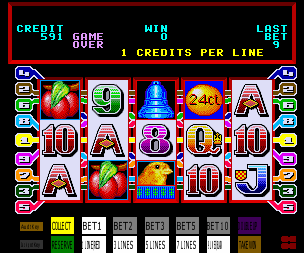## Listed and emulated in MAME !
Info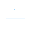(0)
Edit
Upload
### Golden Canaries © 1993 Aristocrat.

A video slot machine with 5 reels and 9 lines.
TECHNICAL INFORMATION
1
Aristocrat 540 MKIV hardware.

MVP cabinet (14 button) layout :
Top row buttons :
Collect
Bet 1 Credit
Bet 2 Credits
Bet 3 Credits
Bet 5 Credits
Bet 10 Credits

Bottom row buttons :
Service
Play 1 Line / Red
Play 3 Lines
Play 5 Lines
Play 7 Lines
Play 9 Lines / Black
Gamble
Take Win

This machine was also released on the MKV hardware (1995), and on the Aristocrat 2.5 Hardware prior to the 540 MKIV.
SCORING
All pays are left to right and/or right to left.

Wild Cat appears on reel 3 and substitutes for all symbols.

Canary : 1 = 2, 2 = 20, 3 = 200, 4 = 1500, 5 = 7500
Granny : 1 = 2, 2 = 10, 3 = 100, 4 = 500, 5 = 5000
Fruit : 2 = 5, 3 = 100, 4 = 300, 5 = 3000
Bell : 2 = 5, 3 = 100, 4 = 200, 5 = 2000
Note : 2 = 5, 3 = 100, 4 = 200, 5 = 2000
Ace : 3 = 30, 4 = 100, 5 = 500
King : 3 = 30, 4 = 100, 5 = 500
Queen : 3 = 15, 4 = 100, 5 = 500
Jack : 3 = 15, 4 = 100, 5 = 500
Ten : 3 = 5, 4 = 50, 5 = 500
Nine : 3 = 5, 4 = 50, 5 = 500
Eight : 3 = 5, 4 = 50, 5 = 500
Egg : 3 = 2, 4 = 10, 5 = 50

Feature : 8 free games are won with a 3, 4 or 5x scattered Egg win. Your overall win from the free games is repeated by the total Canaries which appeared anywhere in the window during the free games. Additional free games can also be won during the feature.
SERIES
SOURCES
Machine's picture.
Game's ROM.